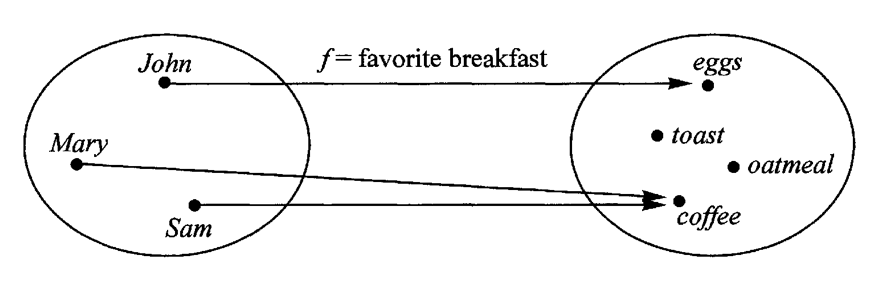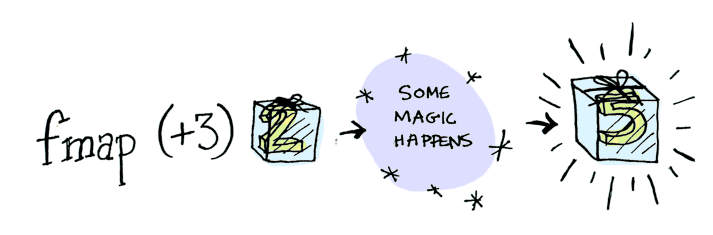#2018 iT 邦幫忙鐵人賽DAY 30
0

## Good Morning, Functional JS (Day 29, functor 函子)

• 目標：是讀懂 FP，能用 code 與人交流，而不是被壓在 FP 的術語大山下喘不過氣。
• 提醒：本文中各種的 FP 小工具，僅為邏輯演示，實際上並不適合在 production 中使用，建議使用 FP library。
• 原文地址：Functional-Light JavaScript

## map 映射Figure from Functional-Light JavaScript

``````var portugueseFood = [
'galinha',
'vaca',
'milho'
]

portugueseFood.map(capitalize)
// ['Galinha', 'Vaca', 'Milho']

``````

1. map(..) 是一個函數，接受一個參數 (mapper function)
2. 傳入 capitalize 為 mapper function
3. map(..) 將每一個元素一一傳入 mapper function
4. 最後將被 mapper function 轉換後元素，放到新的陣列，並返回Picture from Mattias Petter Johansson

``````var increment = v => ++v;
var decrement = v => --v;
var square = v => v * v;

var double = v => v * 2;

var result = [increment,decrement,square]
.map( fn => R.compose( fn, double ) )
.map( fn => fn( 3 ) );

console.log(result)
// [7, 5, 36]
``````

## functor

A functor is something that can be mapped over.

### 學術定義

function 與 functor，儘管都是用來表示映射關係，但兩者目標不同。

functor 是一種範疇，包含了轉換成另一個範疇的映射關係（由外部傳入方法）。• 一個容器，或一個集合
• 包含了值的映射關係（map）
• 轉換後也是另一個 functor

### 實例

``````class Wrapper {
constructor (value) {
this.value = value
}
map (f) {
return new Wrapper(f(this.value))
}
}
``````

``````let something = new Wrapper(2)
console.log(something)
// => { value: 2 }

something.map(function (value) {
return value + 3;
})

// => Wrapper(5)
``````

functor 本身有對外的 API，比如說 map(..)，透過傳入 mapper function，使內部值轉換